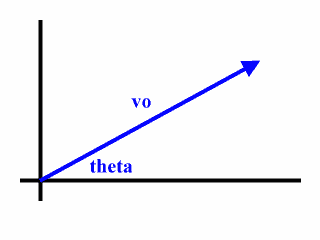# Projectile Motion - Common Questions Calculator

After you have studied the projectile motion material at General Solution In Two Dimensions, No Air Resistance, you may want to to solve an example problem of your own creation. Make up an original velocity vector. Then see if you can find solutions to all the common questions:

• What is the half time of flight?
• What is the total time of flight?
• What is the height of the trajectory?
• What is the range of the trajectory?
• What is the vector displacement at a certain time?
• What is the vector velocity at a certain time?

To get the solution started you must find the x- and y-components of the original velocity vector. That work requires an understanding of vector components and right triangle trigonometry.

The calculator below will help you check your work. It generates a large Web page that appears in its own window, (which you can easily close with a button click). It may take a few seconds to create this Web page, so, be patient after you click the 'Calculate' button.

Consider an object thrown with a velocity as shown in the following diagram:Enter the size and direction, (angle theta in the diagram), for an original velocity below. The calculator will provide values for the following:

• Half time of flight
• Total time of flight
• Height, (maximum y-displacement), for trajectory
• Range, (maximum x-displacement), for the trajectory
• The velocity vector's size and direction, along with its x- and y- component, at the certain time
• The displacement vector's size and direction, along with its x- and y-component, at the certain time

 vo (size): m/s vo (direction): degrees N of E
Certain time: seconds

Custom Search